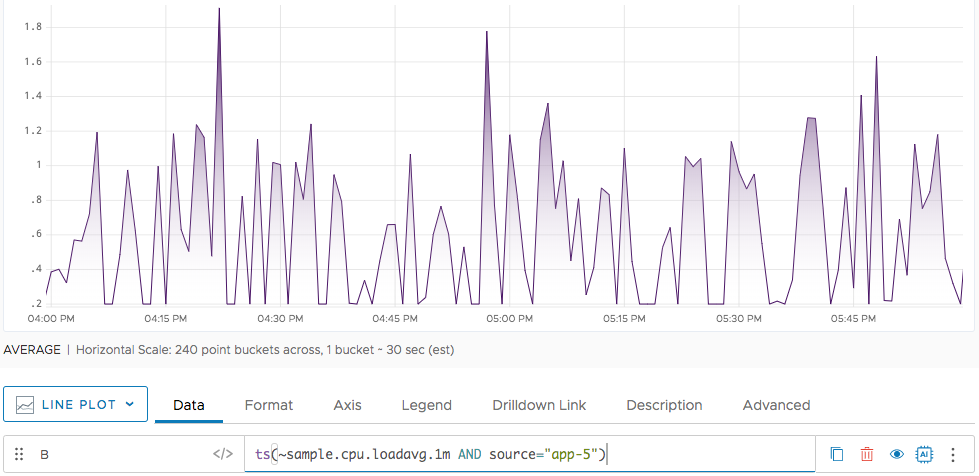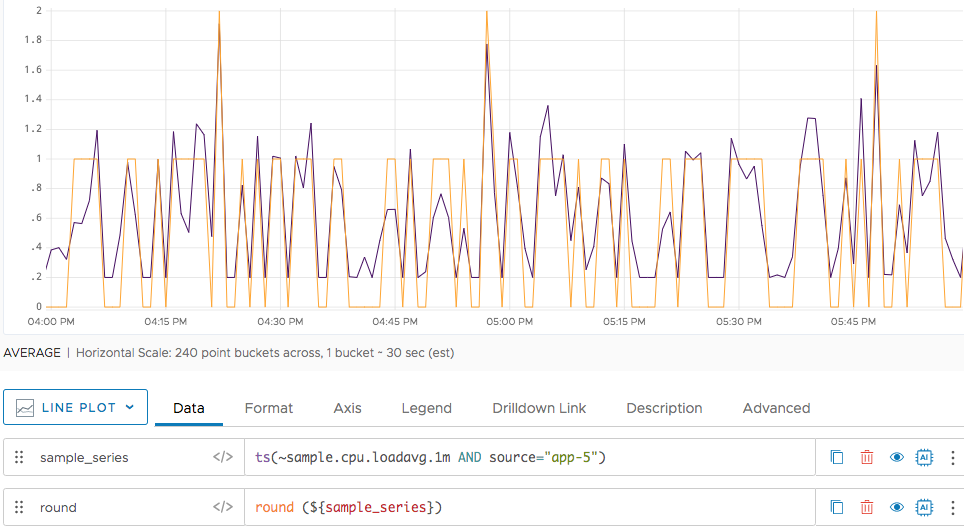Reference to the round() function

## Summary

``````round(<tsExpression>)
round(<toMultiple>, <tsExpression>)
``````

Returns the nearest integer for each data value in the time series described by the expression. Supports rounding toward a number whose multiple you want to round towards.

## Parameters

ParameterDescription
tsExpression Expression that describes the time series to return rounded values for.
toMultiple Number whose multiple you want to round toward (integer or decimal)

## Description

The `round()` function returns the nearest integer for each data value in the time series described by the expression, by mapping any data value with a fractional part to the integer that is closest in value.

`round()` returns a separate series of results for each time series described by the expression.

## Examples

The two different signatures of the function are usually used in different use cases.

### Example 1: Use round() for Precision

One use of `round()` is for achieving precision. For example, suppose that a series `mySeries` returns 0.564, 0.435, 0.777. The math you need to do requires 1 decimal point. You can use `round()` with the `nearestNumber` parameter like this:

``````round(0.5, mySeries)
``````

This call returns `0.5, 0.5. 1.0`.

### Example 2: Visualize round() Results

Here’s a query that returns a time series that reports positive fractional values between .2 and 1.2. Here’s the chart, with the color set to purple.Now we apply `round()` to our original query. Notice that the values below .5 are mapped to 0, and the values between .5 and 1.2 are mapped to 1. The original query is still in purple, the round is set to orange.`ceil()`
`floor()`